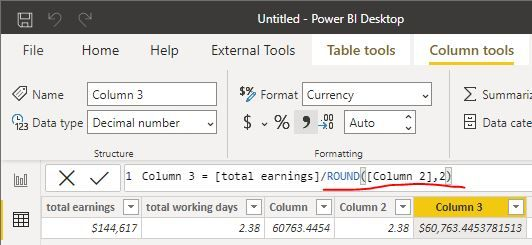cancel
Showing results for
Did you mean:

## Dividing in Power BI Always Wrong

Hello,

In my report, I'm trying to get the charges per day for my sales tickets by taking a column (datatype = currency) containing the total earnings and dividing by another column (datatype = decimal) which has my total working days. However, no matter if I use the DIVIDE function or simply the "/" operator, my results column always renders an incorrect value. I've manually calculated what the total per day should be manually on 3 different devices which gives me the correct answer except for Power BI. For instance, I have a total of \$144,617.00 made with a working days amount of 2.38. Taking the division of that "manually gives me \$60,763.45 but Power BI gives me \$60,891.37 instead. Even creating measures does not work correctly. This is extremely perplexing.

Status: NewCommunity Support

hi

I have tested on my side, it wroks well.and I calculate \$144,617.00/\$60,891.37 = 2.3749999

i think the 2.38 in your dataset is the format of two decimal digits.

so the problem should be this:I used column 2 =2.375009 and keep the format of two decimal digits, Now it shows 2.38

and then divide column, it show result 60891.1377

So just adjust it as below:

Column 3 = [total earnings]/ROUND([Column 2],2)Regards,
Lin
Completed Ideas